24 Feb 2022

Posted on:

24 Feb 2022

1

# Resolved:How to do bitwise operations?

I find it hard to solve question no 17.
Could you explain ?

Instructor
Posted on:

24 Feb 2022

0

Hey Swarntam,

This question is quite tricky and it requires some knowledge on binary numbers and operations. In this answer, I will just explain the relevant components to solving the task but will leave exploring the solution to you :)

First, look up the decimal numbers in binary. You can easily find them through a quick internet search.

Now that you have them, let me explain the bitwise operations that Giles includes in his solution. These are
& (AND)
^ (XOR)
<< (left shift)

The rule for the AND operation (also known as an AND gate) is as follows:
0 & 0 = 0
0 & 1 = 0
1 & 0 = 0
1 & 1 = 1

The rule for the XOR operation (also known as an XOR gate) is as follows:
0 ^ 0 = 0
0 ^ 1 = 1
1 ^ 0 = 1
1 ^ 1 = 0

You can convince yourself that these are true by typing the expression into Python (for example, `1 ^ 1` would give 0 as an output):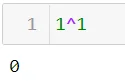If the numbers `a` and `b` are, for example, 5 and 3, then you would perform the AND operation as follows:
0101
0011
-------
0001
where the first column (starting from right to left) consists of 1 and 1 and gives 1 in the end, the second column consists of 0 and 1 and gives 0 in the end, the third column consists of 1 and 0 and gives 0 in the end and finally, the last column consists of 0 and 0 and gives 0 in the end. Converting the result into decimal gives simply 1: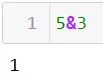The same reasoning is applied for the XOR operation:
0101
0011
-------
0110

which, when converting to base 10, equals 6: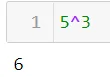The << operation is a bit more tricky. It tells you how many bits you need to shift to the left. Shifting 1 bit to the left would correspond to multiplying the number by 2 to the power of 1, which is 2. Therefore: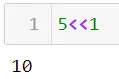The reason this is true is because 5 in binary is 0101. Shifting everything by 1 bit to the left results in 1010, which, in base 10, is just 10.

On the other hand, 5<<2 would multiply 5 by 4 because 2 to the power of 2 is 4. Therefore: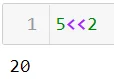This corresponds to shifting everything to the left two times.

Having this knowledge, try to understand what the steps in Giles' solution are. This is a broad topic and, unfortunately, cannot be answered so straightforwardly. Try and explore the bitwise operations yourself. The internet is your friend here! Solve a couple of exercises and then come back to this question again :)

Kind regards,
365 Hristina

Posted on:

24 Feb 2022

0

Thank you for putting so much effort. You guys are amazing.